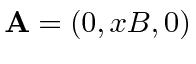## Sample Test Problems

1. A charged particle is in an external magnetic field. The vector potential is given by. What are the constants of the motion? Prove that these are constants by evaluating their commutator with the Hamiltonian.

2. A charged particle is in an external magnetic field. The vector potential is given by. What are the constants of the motion? Prove that these are constants by evaluating their commutator with the Hamiltonian.

3. Gauge symmetry was noticed in electromagnetism before the advent of Quantum Mechanics. What is the symmetry transformation for the wave function of an electron from which the gauge symmetry for EM can be derived?

Jim Branson 2013-04-22